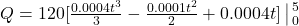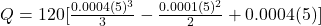## An aluminum “12 gauge” wire has a diameter d of 0.205 centimeters. The resistivity ρ of aluminum is 2.75×10−8 ohm-meters. The electric field

Question

An aluminum “12 gauge” wire has a diameter d of 0.205 centimeters. The resistivity ρ of aluminum is 2.75×10−8 ohm-meters. The electric field in the wire changes with time as E(t)=0.0004t2−0.0001t+0.0004 newtons per coulomb, where time is measured in seconds.

in progress 0
5 months 2021-09-01T10:51:03+00:00 1 Answers 0 views 0

1. Complete Question

An aluminum “12 gauge” wire has a diameter d of 0.205 centimeters. The resistivity ρ of aluminum is 2.75×10−8 ohm-meters. The electric field in the wire changes with time as E(t)=0.0004t2−0.0001t+0.0004 newtons per coulomb, where time is measured in seconds.

I = 1.2 A at time 5 secs.

Find the charge Q passing through a cross-section of the conductor between time 0 seconds and time 5 seconds.

The charge is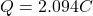Explanation:

From the question we are told that

The diameter of the wire isThe radius of  the wire is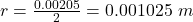The resistivity of aluminum is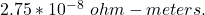The electric field change is mathematically defied as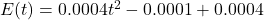Generally the charge is  mathematically represented as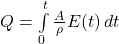Where A is the area which is mathematically represented as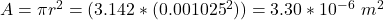So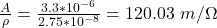Therefore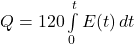substituting values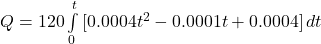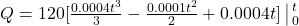From the question we are told that t =  5 sec